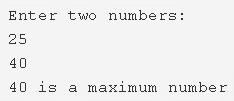# C Program Find Maximum Between Two Numbers | C Programs

C program to find maximum between two numbers – In this article, we will discuss in on the several methods to find maximum between two numbers in C programming along with sample outputs.

Suitable examples and sample programs have also been added so that you can understand the whole thing very clearly. The compiler has also been added with which you can execute it yourself.

The methods used in this piece are as follows:

• Using Standard Method
• Using Macros
• Using Function

As we are discussing in this blog, a maximum number between two numbers is decided based on their absolute value. The signs also matter since negative integers are smaller than positive integers.Given in the photo above, you need to first enter two numbers.

In this case, the two numbers that have been entered are 25 and 40.

It is evident that 40 is greater than 25. Hence, 40 will be printed as the maximum number.

Thus, doing the same in C programming is as follows:

## Using Standard Method

1. Read the entered two numbers and store in the variables a,b.

2) If a>b then print “a is maximum number” otherwise print “b is the maximum number”.

Output:

### Using Macros

1. We are using macros concept. Here #define is the preprocessor. Max is defined as (a>b?a:b). So, In the program max(a,b) will replaced by (a>b?a:b).

2) So In the program max(a,b) will replaced by the conditional operator (a>b?a:b).

3) If a>b then it evaluates ‘a’ otherwise ‘b’.

Output:

#### Using Function

1. The main() calls the maximum(int a, int b) function to find the maximum of two numbers.

2) The function maximum(int a,int b) returns ‘a’ if a>b.Otherwise, it returns ‘b’.

3) Store the returned value in the variable res. Print the res value which is the maximum of two numbers.

Output:
x

## C Program To Print Number Of Days In A Month | Java Tutoring

C program to input the month number and print the number of days in that ...